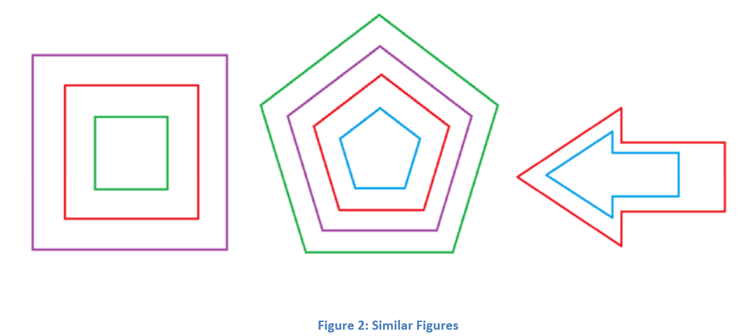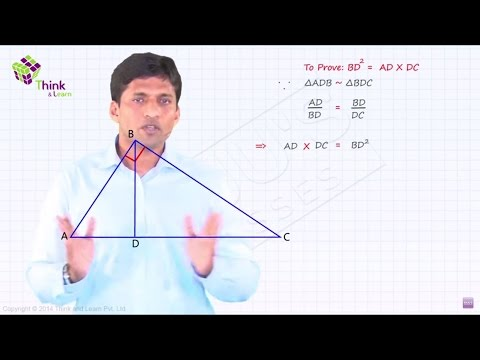Checkout JEE MAINS 2022 Question Paper Analysis : Checkout JEE MAINS 2022 Question Paper Analysis :

# Similar Figures

Similar figures are two figures having the same shape. The objects which are of exactly the same shape and size are known as congruent objects. For example, in real life you will see, both the front wheels of a car, both hands of a person etc. are examples of congruent figures or objects. But the similar shape objects have the same shape but their sizes are different. The symbol is utilised to symbolise similarity.

Certain geometrical shapes or figures are always similar in nature. Consider a circle, if the radius of the circle keeps on changing, its shape still remains the same. Therefore, it can be said that all the circles with different radii are similar to each other. The figure given below represents the concentric circles whose radii are different but all of them are similar. Although these circles have the same shape their sizes are different and therefore these are not congruent.

## Similar Figures Definition

In terms of Maths, when two figures have the same shape but their sizes are different, then such figures are called similar figures. For example, different sized photographs of a person i.e. stamp size, passport size etc. depict the similar objects but are not congruent. In geometry, two similar shapes such as similar triangles, similar rectangles, similar square, are the shapes whose dimensions are in equal or common ratio but the size or length of their sides vary. The common ratio is called the scale factor. Also, the corresponding angles are equal in measure.

If two figures are similar then they are represented by the symbol ‘∼’. Let us say there are two triangles, ABC and PQR, which are similar, then they are represented as;

∆ABC ~ ∆ PQR

Now if the two triangles are similar to each then their corresponding sides should be in proportion. Hence,

• AB/PQ = BC/QR = AC/PR
• ∠A = ∠P, ∠B = ∠Q, ∠C = ∠R

### Similar Figures Area and Volume

If two figures are similar, then their corresponding sides are proportional. Or wen can the ratio of their sides are equal.

Now, if we take the ratio of their surface areas, then it will be equal to the square of ratio of side. The ratio of the volume of two similar figures will be equal to cube of ratio of the lenght of sides.

Note: These are not the surface area and volume of the figures but the ratios.

Hence, based on the statements mentioned above, the scale factors of area and volume can be represented as;

SFA = SF2
SFV = SF3
where SFA is the scale factor of surface area and SFV is the scale factor of volume

### Similar Figures Examples

Consider the figures shown below. All of them are similar to each other as they have the same shape but they are not congruent. It has to be kept in mind that whenever we talk about similar figures, we consider their shapes only, irrespective of their sizes. Thus we can conclude that all congruent figures are similar, but all similar figures are not congruent.If we observe all the figures which are similar, we can see that for any n-sided polygon, the inclination angles of each of the line segments are always the same, irrespective of the size of the figure. Thus for any two n-sided polygons of the same number of sides, it can be said that they are similar if,

1. Corresponding angles of both the polygons are equal, and
2. Corresponding sides of both the polygons are in the same ratio.

It can be said that congruence is a special case of similarity when the ratio of the sides of the figure is 1. In other words, it can be said that when two similar figures become congruent, then their length of corresponding sides becomes equal, as the ratio of their corresponding sides become 1.